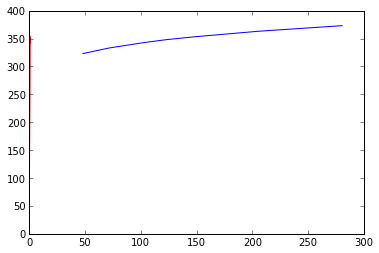Chapter 8 : Phase equilibria¶

Example 8.6¶

In :
# Variables
P1 = 106.; 			#vapour pressure of n-heptane (kPa)
P2 = 74.; 			#vapour pressure of toluene (kPa)
P = 101.3; 			#total pressure (kPa)

# Calculations
x = (P-P2)/(P1-P2)
y = x*(P1/P)

# Results
print 'Composition of liquid heptane is %f mol percent'%(x*100)
print ' Composition of heptane in vapour form is %f mol percent'%(y*100)
Composition of liquid heptane is 85.312500 mol percent
Composition of heptane in vapour form is 89.270731 mol percent

Example 8.7¶

In :
# Variables
P1 = 135.4; 			#vapour pressure of benzene (kPa)
P2 = 54.; 			#vapour pressure of toluene (kPa)
x =  0.5; 			#liquid phase composition

# Calculations
#Using eq. 8.51 (Page no. 332)
P_beg = P2 + (P1-P2)*x;

#At the end
y = 0.5; 			#vapour phase composition
#Using eq. 8.54 (Page no. 333) and rearranging
P_end = (P1*P2)/(P1-y*(P1-P2))

# Results
print 'Pressure at the beginning of the process is %f kPa'%(P_beg)
print ' Pressure at the end of the process is %f kPa'%(P_end)
Pressure at the beginning of the process is 94.700000 kPa
Pressure at the end of the process is 77.208025 kPa

Example 8.8¶

In :
import math
def P1(T):
y1 = math.e**(14.5463 - 2940.46/(T-35.93))  			#vapour pressure of acetone
return y1

def P2(T):
y2 = math.e**(14.2724 - 2945.47/(T-49.15))  			#vapour pressure of acetonitrile
return y2

# Variables
T = 327.; 			#temperature in K
P = 65.; 			#pressure in kPa

# Calculations and Results
P1_s = P1(T)
P2_s = P2(T)
#Using eq. 8.51 (Page no. 332)
x1 = (P-P2_s)/(P1_s-P2_s)
#Using eq. 8.54 (Page no. 333)
y1 = x1*(P1_s/P)
print '(a)'
print ' x1 = %f'%x1
print ' y1 = %f'%y1

#(b). To calculate T and y1
P = 65.; 			#pressure in kPa
x1 = 0.4;

flag = 1.;
T2 = 340.; 			#temperatue (assumed)
while(flag==1):
P1_s = P1(T2)
P2_s = P2(T2)
P_calc = P2_s + x1*(P1_s-P2_s)
if((P_calc-P)<=1):
flag = 0;
else:
T2 = T2-0.8;

y1 = x1*(P1_s/P)
print ' (b)'
print ' Temperature is %f K'%T2
print ' y1 = %f'%y1

#(c). To calculate P and y1
T3 = 327.; 			#temperature in K
x1 = 0.4;

P1_s = P1(T3)
P2_s = P2(T3)
P = P2_s + x1*(P1_s-P2_s)
y1 = x1*(P1_s/P)
print ' (c)'
print ' Pressure is %f kPa'%P
print ' y1 = %f'%y1

#(d). To calculate T and x1
P = 65.; 			#pressure in kPa
y1 = 0.4;

flag = 1.;
T = 340.; 			#assumed temperature (K)
while(flag==1):
P1_s = P1(T)
P2_s = P2(T)
y1_calc = (P1_s*(P-P2_s))/(P*(P1_s-P2_s))
if((y1_calc-y1)>=0.001):
flag = 0;
else:
T = T-2;

x1 = y1*(P/P1_s)
print ' (d)'
print ' Temperature = %f K'%T
print ' x1 = %f'%x1

#(e). To calculate P and x1
T = 327.; 			#temperature (K)
y1 = 0.4;

P1_s = P1(T)
P2_s = P2(T)
#Using eq. 8.54 and 8.51
x1 = (y1*P2_s)/(P1_s-y1*(P1_s-P2_s))
P = x1*(P1_s/y1)
print ' (e)'
print ' Pressure = %f kPa'%P
print ' x1 = %f'%x1

#(f). To calculate fraction of the system is liquid and vapour in equilibrium
T = 327.; 			#temperature (K)
P = 65.; 			#pressure (kPa)
y1 = 0.7344;

P1_s = P1(T)
P2_s = P2(T)
x1 = (P-P2_s)/(P1_s-P2_s)
#Let f be the fraction of the mixture that is liquid
#Applying acetone balance
f = (0.7-y1)/(x1-y1)
print ' (f)'
print ' Fraction of mixture that is liquid is %f percent'%(f*100)
(a)
x1 = 0.560806
y1 = 0.734393
(b)
Temperature is 330.400000 K
y1 = 0.588617
(c)
Pressure is 57.633467 kPa
y1 = 0.590765
(d)
Temperature = 334.000000 K
x1 = 0.240940
(e)
Pressure = 50.093199 kPa
x1 = 0.235402
(f)
Fraction of mixture that is liquid is 19.816333 percent

Example 8.9¶

In :
%matplotlib inline

# Variables
P = 101.3; 			#total pressure over the system (kPa)
T = [371.4, 378, 383, 388, 393, 398.6];
Pa = [101.3 ,125.3, 140.0, 160.0 ,179.9, 205.3];
Pb = [44.4 ,55.6 ,64.5, 74.8, 86.6 ,101.3];
xa = [0,0,0,0,0,0]
ya = [0,0,0,0,0,0]

# Calculations
#To construct boiling point and equilibrium point diagram
for i in range(6):
xa[i] = (P-Pb[i])/(Pa[i]-Pb[i]) 			#Using eq. 8.51
ya[i] = xa[i]*(Pa[i]/P )			#Using eq. 8.54

from matplotlib.pyplot import *
#(a).
#To construct boiling point diagram
plot(xa,T)
plot(ya,T)
#title("Boiling Point diagram xa and ya Temperature"
#(b).
#To construct the equilibrium diagram
plot(ya,xa)
#title("Equilibrium Diagram","xa","ya"
show()
#(c).

# Results
print '(c). The given subpart is theoretical and does not involve any numerical computation'
Populating the interactive namespace from numpy and matplotlib
WARNING: pylab import has clobbered these variables: ['draw_if_interactive']
%pylab --no-import-all prevents importing * from pylab and numpy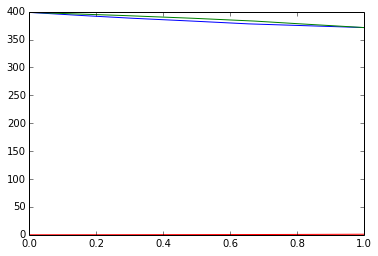(c). The given subpart is theoretical and does not involve any numerical computation

Example 8.11¶

In :
# Variables
x1 = 46.1/100; 			#mole percent of A
P = 101.3; 			#total pressure of system (kPa)
P1_s = 84.8; 			#vapour pressure of component A (kPa)
P2_s = 78.2; 			#vapour pressure of component B (kPa)

# Calculations
#To calculate van Laar constants
gama1 = P/P1_s;
gama2 = P/P2_s;
x2 = 1-x1;
import math
#van Laar constants:
#Using eq. 8.69 (Page no. 348)
A = math.log (gama1)*(1 + (x2*math.log(gama2))/(x1*math.log(gama1)))**2;
B = math.log (gama2)*(1 + (x1*math.log(gama1))/(x2*math.log(gama2)))**2;

# Results
print 'van Laar constants are:'
print ' A = %f'%A
print ' B = %f'%B
van Laar constants are:
A = 1.298059
B = 0.652282

Example 8.12¶

In :
# Variables
x2 = 0.448; 			#mole fraction of ethanol
P = 101.3; 			#total pressure (kPa)
P1_s = 68.9; 			#Vapour pressure of benzene (kPa)
P2_s = 67.4; 			#vapour pressure of ethanol (kPa)

# Calculations
#To calculate activity coeffecients in a solution containing 10% alcohol
x1 = 1-x2;
gama1 = P/P1_s;
gama2 = P/P2_s;
import math
#Using eq. 8.69 (Page no. 348)
#van Laar constants:
A = math.log(gama1)*(1 + (x2*math.log(gama2))/(x1*math.log(gama1)))**2;
B = math.log(gama2)*(1 + (x1*math.log(gama1))/(x2*math.log(gama2)))**2;

#For solution containing 10% alcohol
x2 = 0.1;
x1 = 1-x2;
ln_g1 = (A*x2**2)/(((A/B)*x1+x2)**2)
ln_g2 = (B*x1**2)/((x1+(B/A)*x2)**2)
gama1 = math.e**ln_g1;
gama2 = math.e**ln_g2;

# Results
print 'Activity coeffecients:'
print ' For component 1: %f'%gama1
print ' For component 2: %f'%gama2
Activity coeffecients:
For component 1: 1.025516
For component 2: 4.141567

Example 8.13¶

In :
# Variables
x2 = 0.585; 			#mol fraction of hydrazine
P = 101.3; 			#total pressure of system (kPa)
P2_s = 124.76; 			#vapour pressure of hydrazine (kPa)

# Calculations
#To calculate equilibrium vapour composition for solution containing 20% (mol) hydrazine
x1 = 1-x2;
P1_s = 1.6*P2_s; 			#vapour pressure of water (kPa)
gama1 = P/P1_s;
gama2 = P/P2_s;

import math
#Using eq. 8.69 (Page no. 348)
#van Laar constants:
A = math.log(gama1)*(1 + (x2*math.log(gama2))/(x1*math.log(gama1)))**2;
B = math.log(gama2)*(1 + (x1*math.log(gama1))/(x2*math.log(gama2)))**2;

#For solution containing 20% hydrazine
x2 = 0.2;
x1 = 1-x2;
ln_g1 = (A*x2**2)/(((A/B)*x1+x2)**2)
ln_g2 = (B*x1**2)/((x1+(B/A)*x2)**2)
gama1 = math.e**ln_g1;
gama2 = math.e**ln_g2;

#Using eq. 8.47 (Page no. 325) for components 1 and 2 and rearranging
alpha = 1.6; 			#alpha = P1_s/P2_s
y1 = 1./(1 + (gama2*x2)/(gama1*x1*alpha))
y2 = 1-y1;

# Results
print 'Equilibrium vapour composition for solution containing 20 mol percent hydrazine'
print ' Hydrazine is %f percent'%(y2*100)
print ' Water is %f percent'%(y1*100)
Equilibrium vapour composition for solution containing 20 mol percent hydrazine
Hydrazine is 5.279270 percent
Water is 94.720730 percent

Example 8.15¶

In :
#Given:
x1 = 0.047; 			#mol fraction of isopropanol
P1 = 91.11; 			#vapour pessure of pure propanol (kPa)
P = 91.2; 			#toatl pressure of system (kPa)
P2 = 47.36; 			#vapour pressure of water (kPa)

#van Laar consatnts:
A = 2.470;
B = 1.094;

#To determine the total pressure:
x2 = 1-x1;
#Using eq. 8.68 (Page no. 348)
ln_g1 = (A*x2**2)/(((A/B)*x1 + x2)**2);
ln_g2 = (B*x1**2)/((x1 + (B/A)*x2)**2);
gama1 = math.e**ln_g1;
gama2 = math.e**ln_g2;
#Total pressure:
P_tot = (gama1*x1*P1) + (gama2*x2*P2);

# Results
if(P==P_tot):
print 'This is equal to total pressure'
else:
print 'This is less than the total pressure. This error must have been caused by air leak'

This is less than the total pressure. This error must have been caused by air leak

Example 8.16¶

In :
# Variables
P1 = 24.62; 			#vapour pressure of cyclohexane (kPa)
P2 = 24.41; 			#vapour pressure of benzene (kPa)
from numpy import array
import math
x1 = array([0, 0.2, 0.4, 0.6, 0.8, 1.0])
x2 = 1-x1;
g1 = [0,0,0,0,0,0]
g2 = [0,0,0,0,0,0]
P = [0,0,0,0,0,0]
y1 = [0,0,0,0,0,0]

# Calculations
for i in range(6):
g1[i] = math.e**(0.458*x2[i]**2) 			#activity coeffecient for component 1
g2[i] = math.e**(0.458*x1[i]**2 )			#activity coeffecient for component 2
P[i] = (g1[i]*x1[i]*P1) + (g2[i]*x2[i]*P2) 			#total pressure (kPa)
y1[i] = (g1[i]*x1[i]*P1)/P[i];

from matplotlib.pyplot import *

# Results
#To construct P-x-y diagram
plot(x1,P)
plot(y1,P)
#title("P-x-y Diagram","x1 and y1","Pressure"
show()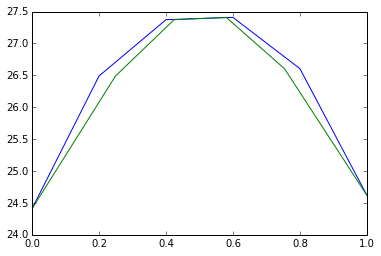Example 8.17¶

In :
# Variables
P = 40.25; 			#total pressure (kPa)
y1 = 0.566; 			#mol fraction of benzene in vapour phase
x1 = 0.384; 			#mol fraction of benzene in liquid state
P1 = 29.6; 			#vapour pressure of benzene (kPa)
P2 = 22.9; 			#vapour pressure of ethanol (kPa)

#To determine the composition and total pressure of azeotrope
x2 = 1-x1;
y2 = 1-y1;

# Calculations
#Using eq. 8.47 (Page no. 325)
#Activity coeffecients:
g1 = (y1*P)/(x1*P1)
g2 = (y2*P)/(x2*P2)

import math
#Using eq. 8.69 (Page no. 348)
#van Laar constants:
A = math.log(g1)*((1 + (x2*math.log(g2))/(x1*math.log(g1)))**2)
B = math.log(g2)*((1 + (x1*math.log(g1))/(x2*math.log(g2)))**2)

#Assuming azeotropic comp. (for hit and trial method)
x1 = 0.4;
flag = 1.;
while(flag==1):
x2 =1-x1;
ln_g1 = (A*x2**2)/(((A/B)*x1 + x2)**2)
ln_g2 = (B*x1**2)/((x1 + (B/A)*x2)**2)
g1 = math.e**ln_g1;
g2 = math.e**ln_g2;
P_1 = g1*P1;
P_2 = g2*P2;
if((P_1-P_2)<=1) and ((P_1-P_2)>=-1):
flag = 0;
else:
x1 = x1+0.1;

# Results
print 'Azeotropic compositon of benzene is %i percent'%(x1*100)
print ' Total pressure of azeotrope is %f kPa'%((P_1+P_2)/2)
Azeotropic compositon of benzene is 60 percent
Total pressure of azeotrope is 40.858067 kPa

Example 8.19¶

In :
# Variables
a12 = 1225.31; 			#(J/mol)
a21 = 6051.01; 			#(J/mol)
V1 = 74.05*10**-6; 			#(m**3/mol)
V2 = 18.07*10**-6; 			#(m**3/mol)

R = 8.314; 			#ideal gas constant
T = 349; 			#temperature in K

# Calculations
#Antoine Equation:
#Vapour pressure of 1st element
def P1(T):
y1 = math.e**(14.39155-(2795.817/(T-43.198)))
return y1

#Vapour pressure of 2nd element
def P2(T):
y2 = math.e**(16.26205-(3799.887/(T-46.854)))
return y2

#To calculate equilibrium pressure and composition
#Using eq. 8.73 (Page no. 350)
#Wilson Parameters:
W12 = (V2/V1)*math.e**(-a12/(R*T));
W21 = (V1/V2)*math.e**(-a21/(R*T));
import math
#Using Antoine equation
P1_s = P1(T);
P2_s = P2(T);

#(a). Composition of vapour in equilibrium
x1 = 0.43;
x2 = 1-x1;

#Using eq. 8.72 (Page no. 350)
#Wilson equations:
#Activity coeffecient of 1st component
def g_1(n1,n2): 			#n1 is mol fraction of 1 and n2 is for 2
y3 = math.e**(-math.log(n1 + W12*n2) + n2*((W12/(n1+W12*n2))-(W21/(W21*n1+n2))));
return y3

#Activity coeffecint of 2nd component
def g_2(n1,n2):
y4 = math.e**(-math.log(n2 + W21*n1) - n1*((W12/(n1+W12*n2))-(W21/(W21*n1+n2))));
return y4

#Activity coeffecients:
g1 = g_1(x1,x2);
g2 = g_2(x1,x2);

P = (g1*x1*P1_s) + (g2*x2*P2_s);
y1 = (g1*x1*P1_s)/P;

# Results
print '(a).'
print ' Equilibrium pressure is %f kPa'%P
print ' Composition of acetone vapour in equilibrium is %f'%y1

#(b). Composition of liquid in equilibrium
y1 = 0.8;
y2 = 1-y1;
g1 = 1; g2 = 1; 			#assumed activity coeffecients
P_as = 1/((y1/(g1*P1_s)) + (y2/(g2*P2_s)));

#Hit and trial method:
flag = 1;
while(flag==1):
x1 = (y1*P_as)/(g1*P1_s);
x2 = 1-x1;
g1 = g_1(x1,x2);
g2 = g_2(x1,x2);
P_calc = 1/((y1/(g1*P1_s)) + (y2/(g2*P2_s)));
if((P_calc-P_as)<=1) and ((P_calc-P_as)>=-1):
flag = 0;
else:
P_as = P_calc;

print ' (b).'
print ' Equilibrium Pressure is %f kPa'%P_calc
print ' Composition of acetone in liquid in equilibrium is %f'%x1
(a).
Equilibrium pressure is 162.828251 kPa
Composition of acetone vapour in equilibrium is 0.795360
(b).
Equilibrium Pressure is 164.488565 kPa
Composition of acetone in liquid in equilibrium is 0.456817

Example 8.20¶

In :
# Variables
P = 101.3; 			#total pressure of system (kPa)
T = 337.5; 			#temperature in K
x1 = 0.842;

#Antoine constants
#For methanol(1)
A1 = 16.12609;
B1 = 3394.286;
C1 = 43.2;

#For methyl ethyl ketone (2)
A2 = 14.04357;
B2 = 2785.225;
C2 = 57.2;
import math

# Calculations
#To determine parameters in Wilson's equation
P1_s = math.e**(A1-(B1/(T-C1)))
P2_s = math.e**(A2-(B2/(T-C2)))
x2 = 1-x1;
g1 = P/P1_s;
g2 = P/P2_s;

#Using eq. 8.72 and rearranging:
def Wils(n): 			#n is the Wilson's parameter W12
y1 = (((g1*x2)/(1-(n*x1/(x1+n*x2))+(x1/x2)*math.log(g1*(x1+n*x2))))**(x2/x1))*(g1*(x1+n*x2))
return y1

flag = 1;
W12 = 0.5; 			#assumed value
while(flag==1):
res = Wils(W12)
if ((res-1)>=-0.09):
flag = 0;
else:
W12 = W12+0.087;

#For 2nd Wilson parameter:
#Using eq. 8.72 and rearranging:
k = math.log(g1*(x1+W12*x2))/x2 - (W12/(x1+W12*x2))
W21 = (-k*x2)/(1+k*x1)

# Results
print "wilson parameters are: %f, %f"%(W12,W21)
wilson parameters are: 0.935000, 0.470758

Example 8.21¶

In :

from numpy import *

# Variables
P = 101.3; 			#total pressure in kPa
T = [333, 343, 353, 363]; 			#temperatures(K)
Pa = [81.97 ,133.29 ,186.61, 266.58]; 			#Partial pressure of component A (kPa)
Pb = [49.32 ,73.31, 106.63, 166.61]; 			#Partial pressure of component B (kPa)
Pc = [39.32 ,62.65, 93.30, 133.29]; 			#Partial pressure of component C (kPa)
xa = 0.45; 			#mole fraction of methanol
xb = 0.3; 			#mole fraction of ethanol

# Calculations and Results
xc = 1-xa-xb; 			#mole fraction of propanol

#To calculate bubble and dew point and the composition
#(a). To calculate bubble point and vapour composition
from matplotlib.pyplot import *
plot(T,Pa);
plot(T,Pb);
plot(T,Pc);
#title(" ","Temperature","Vapour pressures");
#legend("Pa","Pb","Pc");

#Using eq. 8.84 (Page no. 362)
#At bubble temperature, sum(yi) = sum((xi*Pi)/P) = 1
sum_y = [0,0,0,0]
for i in range(4):
sum_y[i] = (xa*Pa[i])/P + (xb*Pb[i])/P + (xc*Pc[i])/P;

Tb = interp(1,sum_y,T); 			#obtaining temperature at which sum (yi) = 1

#Obtaining vapour pressures at bubble temperature
Pb1 = interp(Tb,T,Pa);
Pb2 = interp(Tb,T,Pb);
Pb3 = interp(Tb,T,Pc);

#Calculating equilibrium vapour composition
ya = (xa*Pb1*100)/P;
yb = (xb*Pb2*100)/P;
yc = (xc*Pb3*100)/P;

print '(a).'
print ' The bubble temperature is %f K'%Tb
print ' The equilibrium vapour contains %f methanol, %f ethanol and %f propanol'%(ya,yb,yc)

#(b). The dew point and liquid composition
#Vapour phase compositions at dew point
ya = 0.45; 			#methanol
yb = 0.30; 			#ethanol
yc = 0.25; 			#propanol

sum_x = zeros(4)
#At dew point, sum(xi) = sum ((yi*P)/Pi) = 1
for i in range(4):
sum_x[i] = (ya*P)/Pa[i] + (yb*P)/Pb[i] + (yc*P)/Pc[i];

Td = interp(1,sum_x,T); 			#obtaining temperature at which sum (xi) = 1

#Obtaining vapour pressures at dew temperature
Pd1 = interp(Td,T,Pa);
Pd2 = interp(Td,T,Pb);
Pd3 = interp(Td,T,Pc);

#Calculating liquid composition
xa = (ya*P*100)/Pd1;
xb = (yb*P*100)/Pd2;
xc = (yc*P*100)/Pd3;

print ' (c).'
print ' The dew point is %f K'%Td
print ' At dew point liquid contains %f methanol, %f ethanol and %f propanol'%(xa,xb,xc)
(a).
The bubble temperature is 343.879659 K
The equilibrium vapour contains 61.294328 methanol, 22.578783 ethanol and 16.126889 propanol
(c).
The dew point is 363.000000 K
At dew point liquid contains 17.099932 methanol, 18.240202 ethanol and 18.999925 propanol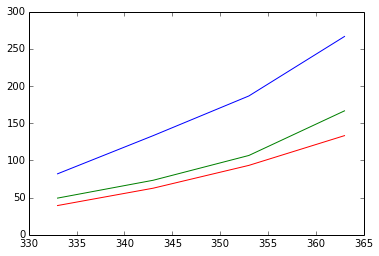Example 8.22¶

In :
#Given:

P = 1447.14; 			#pressure of the system (kPa)
x = [0.25 ,0.4, 0.35]; 			#composition of the components
T = [355.4 ,366.5]; 			#assumed temperatures (K)
K1 = [2.00, 0.78 ,0.33]; 			#value of Ki at 355.4 K
K2 = [2.30, 0.90 ,0.40]; 			#value of Ki at 366.5 K

# Calculation and Result

Kx = [0, 0];
for i in range(3):
Kx = Kx+K1[i]*x[i];
Kx = Kx+K2[i]*x[i];

Tb = interp(1,Kx,T);

#At Tb K, from Fig. 13.6 of Chemical Engineer's Handbook
Kb = [2.12 ,0.85 ,0.37]

#Calculation of vapour composition
y1 = Kb*x*100;
y2 = Kb*x*100;
y3 = Kb*x*100;

print '(a).'
print ' The bubble point temperature is %f K'%Tb
print ' At bubble point vapour contains %f percent propane, %f percent butane and %f percent pentane'%(y1,y2,y3)

#(b). The dew point temperature and composition of the liquid
T = [377.6 ,388.8]; 			#assumed temperatures (K)
y = [0.25, 0.40, 0.35]; 			#vapour composition at dew point
K1 = [2.6, 1.1, 0.5]; 			#at 377.6 K
K2 = [2.9, 1.3, 0.61]; 			#at 388.8 K

#At dew point, sum(yi/Ki) = 1
Ky = [0, 0];
for i in range(3):
Ky = Ky + y[i]/K1[i];
Ky = Ky + y[i]/K2[i];

Td = interp(1,Ky,T);

#At Td K,
Kd = [2.85, 1.25, 0.59];

#Calculation of liquid composition
x1 = y*100/Kd;
x2 = y*100/Kd;
x3 = y*100/Kd;

print ' (b).'
print ' The dew point temperature is %f K'%Td
print ' Liquid at dew point contains %f percent propane, %f percent butane and %f percent pentane'%(x1,x2,x3)

#(c). Temperature and composition when 45% of initial mixture is vaporised
#Basis:
F = 100;
V = 45;
L = 55;

#For the given condition eq. 8.91 (Page no. 364) is to be satisfied
#sum(zi/(1+ L/(VKi))) = 0.45

z = [0.25, 0.4, 0.35];
T = [366.5 ,377.6]; 			#assumed temperatures
K1 = [2.3 ,0.9 ,0.4]; 			#at 366.5 K
K2 = [2.6 ,1.1 ,0.5]; 			#at 377.6 K

Kz = [0 ,0];
for i in range(3):
Kz = Kz + z[i]/(1 + L/(V*K1[i]));
Kz = Kz + z[i]/(1 + L/(V*K2[i]));

#The required temperature is T3
T3 = interp(.45,Kz,T);

#At T3 K
K3 = [2.5, 1.08, 0.48];

#Calculating liquid and vapour compositions
for i in range(3):
y[i] = (z[i]/(1 + L/(V*K3[i])))/0.45;
x[i] = ((F*z[i]) - (V*y[i]))/L;
print (x[i]);

print ' (c).'
print ' The equilibrium temperature is %f K'%T3
print ' Liquid composition in equilibrium is %f percent propane, %f percent butane \
and %f percent pentane'%(x*100,x*100,x*100)
print ' Vapour composition in equilibrium is %f percent propane, %f percent butane \
and %f percent pentane'%(y*100,y*100,y*100);
(a).
The bubble point temperature is 360.855932 K
At bubble point vapour contains 53.000000 percent propane, 34.000000 percent butane and 12.950000 percent pentane
(b).
The dew point temperature is 388.800000 K
Liquid at dew point contains 8.771930 percent propane, 32.000000 percent butane and 59.322034 percent pentane
0.149253731343
0.3861003861
0.456919060052
(c).
The equilibrium temperature is 374.651845 K
Liquid composition in equilibrium is 14.925373 percent propane, 38.610039 percent butane and 45.691906 percent pentane
Vapour composition in equilibrium is 37.313433 percent propane, 41.698842 percent butane and 21.932115 percent pentane

Example 8.23¶

In :
from numpy import array

# Variables
P = 101.3; 			#total pressure (kPa)
x1 = array([0.003, 0.449, 0.700, 0.900])
y1 = array([0.432, 0.449, 0.520, 0.719])
P1 = [65.31 ,63.98, 66.64, 81.31]; 			#(kPa)
P2 = [68.64 ,68.64, 69.31, 72.24]; 			#(kPa)

import math

# Calculations
#To test whether the given data are thermodynamically consistent or not
x2 = 1-x1;
y2 = 1-y1;
g1= [0,0,0,0]
g2 = [0,0,0,0]
c=[0,0,0,0]
import math
for i in range(4):
g1[i] = (y1[i]*P)/(x1[i]*P1[i])
g2[i] = (y2[i]*P)/(x2[i]*P2[i])
c[i] = math.log(g1[i]/g2[i]) 			#k = ln (g1/g2)
from matplotlib.pyplot import *
plot(x1,c)
#a = get("current_axes"
#set(a,"x_location","origin"

# Results
show()
#As seen from the graph net area is not zero
print 'The given math.experimental data do not satisfy the Redlich-Kistern criterion'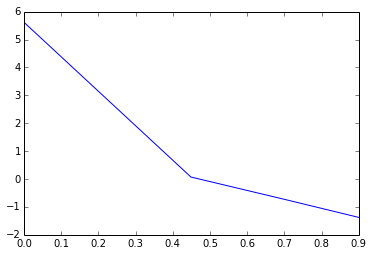The given math.experimental data do not satisfy the Redlich-Kistern criterion

Example 8.25¶

In :
# Variables
x1 = [0.0331, 0.9652]; 			#composition of chloroform
P = [40.84 ,84.88]; 			#total pressure for system (kPa)
P1 = 82.35; 			#vapour pressure of chloroform at 328 K (kPa)
P2 = 37.30; 			#vapour pressure of acetone at 328 K (kPa)

# Calculations
#To estimate the constants in Margules equation
#Using eq. 8.103 and 8.104 (Page no. 375)
g1_inf = (P-(1-x1)*P2)/(x1*P1)
g2_inf = (P-(x1*P1))/((1-x1)*P2)

import math
A = math.log(g1_inf)
B = math.log(g2_inf)

# Results
print 'Margules constants are:'
print ' A = %f'%A
print ' B = %f'%B
Margules constants are:
A = 0.560560
B = 1.424762

Example 8.26¶

In :
# Variables
x1 = [0., 0.033, 0.117, 0.318, 0.554, 0.736, 1.000]; 			#liquid composition of acetone
pp1 = [0.,25.33, 59.05, 78.37, 89.58,94.77, 114.63]; 			#partial pressure of acetone (kPa)
Pw = 19.91; 			#vapour pressure of water at 333 K (kPa)
k = [0,0,0,0,0,0,0]

# Calculations
for i in range(1,6):
k[i] = x1[i]/((1.-x1[i])*pp1[i])

k = 0.1; 			#k(7) should tend to infinity
from matplotlib.pyplot import *
plot(pp1,k)
show()
#From graph% area gives the integration and hence partiaal pressure of water is calculated
pp2 = [19.91, 19.31, 18.27, 16.99, 15.42, 13.90, 0];

# Results
print "The results are:"
print '  Acetone composition      Partial pressure of water'
for i in range(7):
print '       %f                       %f'%(x1[i],pp2[i])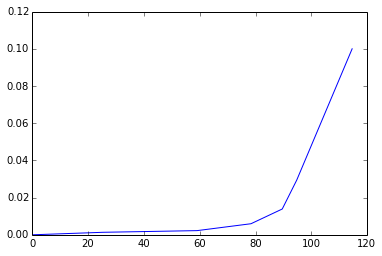The results are:
Acetone composition      Partial pressure of water
0.000000                       19.910000
0.033000                       19.310000
0.117000                       18.270000
0.318000                       16.990000
0.554000                       15.420000
0.736000                       13.900000
1.000000                       0.000000

Example 8.27¶

In :
# Variables
P = 93.30; 			#total pressure in kPa
T1 = 353.; 			#(K)
T2 = 373.; 			#(K)
Pa1 = 47.98; 			#Vapour pressure of water at 353 K (kPa)
Pb1 = 2.67; 			#Vapour pressure of liquid at 353 K (kPa)
Pa2 = 101.3; 			#Vapour pressure of water at 373 K (kPa)
Pb2 = 5.33; 			#Vapour pressure of liquid at 373 K (kPa)

# Calculations and Results
#To calculate under three phase equilibrium:
#(a). The equilibrium temperature
P1 = Pa1+Pb1; 			#sum of vapour pressures at 353 K
P2 = Pa2+Pb2; 			#at 373 K

#Since vapour pressure vary linearly with temperature% so T at which P = 93.30 kPa
T = T1 + ((T2-T1)/(P2-P1))*(P-P1)
print '(a). The equilibrium temperature is %f K'%T

#(b). The composition of resulting vapour
#At equilibrium temp:
Pa = 88.5; 			#vapour pressure of water (kPa)
Pb = 4.80; 			#vapour pressure of liquid (kPa)

#At 3-phase equilibrium% ratio of mol fractions of components is same as the ratio of vapour pressures
P = Pa+Pb; 			#sum of vapour pressures
y = Pa/P; 			#mole fraction of water
print ' The vapour contains %f mol percent water vapour'%(y*100)
(a). The equilibrium temperature is 368.237585 K
The vapour contains 94.855305 mol percent water vapour

Example 8.28¶

In :
# Variables
T = [323 ,333, 343, 348, 353, 363, 373]; 			#temperatures (K)
P2 = [12.40 ,19.86, 31.06, 37.99, 47.32, 70.11, 101.3]; 			#vapour pressure for benzene (kPa)
P1 = [35.85 ,51.85, 72.91, 85.31, 100.50, 135.42, 179.14]; 			#vapour pressure for water (kPa)
Tb = 353.1; 			#boiling temperature (K)
Pb = 101.3; 			#boiling pressure (kPa)

# Calculations and Results
#To prepare temperature composition diagram
#To find three phase temperature
P = [0,0,0,0,0,0,0]
for i in range(7):
P[i] = P1[i] + P2[i];
from matplotlib.pyplot import *
plot(P,T)
#From graph, at P = 101.3 kPa..
T_ = 340.; 			#three phase temperature

#At three phase temperature
P1_ = 71.18; 			#(kPa)
P2_ = 30.12; 			#(kPa)
xb_ = P1_/Pb; 			#mol fraction of benzene at triple point

#For the dew point curve
#For curve BE in temp range from 342 to 373 K
y1 = [0,0,0,0,0,0,0]
for i in range(2,7):
y1[i] = 1-(P2[i]/Pb )

#xset('window',1
T1 = [0,0,0,0,0,0,0]
y1_ = [0,0,0,0,0,0,0]
T1 = 342
y1_ = 0.7;
for i in range(1,6):
T1[i] = T[i+1];
y1_[i] = y1[i+1];

plot(y1_,T1)
y2 = [0,0,0,0,0,0,0]
#For the curve Ae in the temp range of 342 K to 353.1 K
for i in range(2,5):
y2[i] = P1[i]/Pb;

T2 = [0,0,0,0,0,0,0]
y2_ = [0,0,0,0,0,0,0]
T2 = 342.;
y2_ = 0.7;
for i in range(1,4):
T2[i] = T[i+1]
y2_[i] = y2[i+1]

plot(y2_,T2)
#axhspan(0.25,0.75,facecolor='0.5'
#title("Temperature Composition diagram","xa,ya","Temperature"
show()